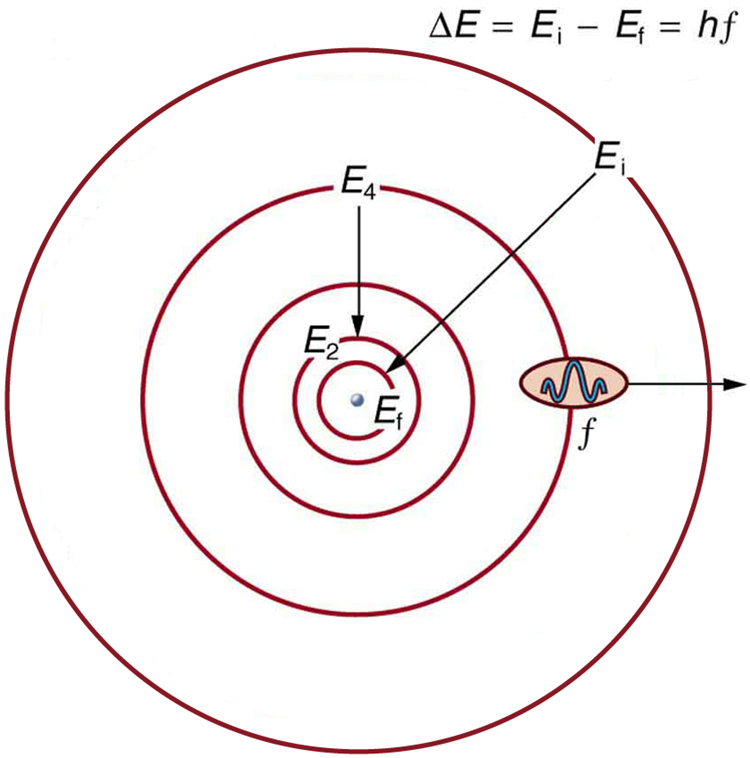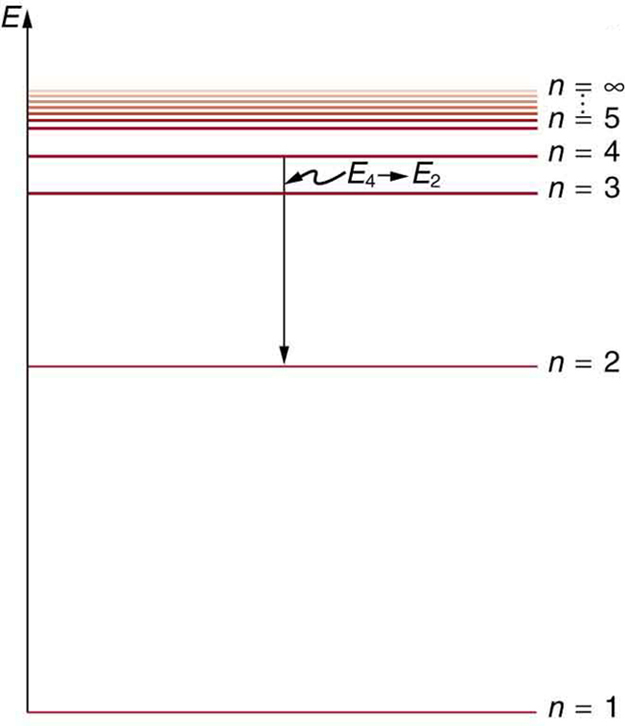# 30.3 Bohr’s theory of the hydrogen atom  (Page 3/14)

 Page 3 / 14

## Bohr’s solution for hydrogen

Bohr was able to derive the formula for the hydrogen spectrum using basic physics, the planetary model of the atom, and some very important new proposals. His first proposal is that only certain orbits are allowed: we say that the orbits of electrons in atoms are quantized . Each orbit has a different energy, and electrons can move to a higher orbit by absorbing energy and drop to a lower orbit by emitting energy. If the orbits are quantized, the amount of energy absorbed or emitted is also quantized, producing discrete spectra. Photon absorption and emission are among the primary methods of transferring energy into and out of atoms. The energies of the photons are quantized, and their energy is explained as being equal to the change in energy of the electron when it moves from one orbit to another. In equation form, this is

$\Delta E=\text{hf}={E}_{i}-{E}_{f}.$

Here, $\Delta E$ is the change in energy between the initial and final orbits, and $\text{hf}$ is the energy of the absorbed or emitted photon. It is quite logical (that is, expected from our everyday experience) that energy is involved in changing orbits. A blast of energy is required for the space shuttle, for example, to climb to a higher orbit. What is not expected is that atomic orbits should be quantized. This is not observed for satellites or planets, which can have any orbit given the proper energy. (See [link] .)The planetary model of the atom, as modified by Bohr, has the orbits of the electrons quantized. Only certain orbits are allowed, explaining why atomic spectra are discrete (quantized). The energy carried away from an atom by a photon comes from the electron dropping from one allowed orbit to another and is thus quantized. This is likewise true for atomic absorption of photons.

[link] shows an energy-level diagram    , a convenient way to display energy states. In the present discussion, we take these to be the allowed energy levels of the electron. Energy is plotted vertically with the lowest or ground state at the bottom and with excited states above. Given the energies of the lines in an atomic spectrum, it is possible (although sometimes very difficult) to determine the energy levels of an atom. Energy-level diagrams are used for many systems, including molecules and nuclei. A theory of the atom or any other system must predict its energies based on the physics of the system.An energy-level diagram plots energy vertically and is useful in visualizing the energy states of a system and the transitions between them. This diagram is for the hydrogen-atom electrons, showing a transition between two orbits having energies E 4 size 12{E rSub { size 8{4} } } {} and E 2 size 12{E rSub { size 8{2} } } {} .

Bohr was clever enough to find a way to calculate the electron orbital energies in hydrogen. This was an important first step that has been improved upon, but it is well worth repeating here, because it does correctly describe many characteristics of hydrogen. Assuming circular orbits, Bohr proposed that the angular momentum $L$ of an electron in its orbit is quantized , that is, it has only specific, discrete values. The value for $L$ is given by the formula

what is physics
what are the basic of physics
faith
tree physical properties of heat
tree is a type of organism that grows very tall and have a wood trunk and branches with leaves... how is that related to heat? what did you smoke man?
what are the uses of dimensional analysis
Dimensional Analysis. The study of relationships between physical quantities with the help of their dimensions and units of measurements is called dimensional analysis. We use dimensional analysis in order to convert a unit from one form to another.
Emmanuel
meaning of OE and making of the subscript nc
Negash
kinetic functional force
what is a principal wave?
A wave the movement of particles on rest position transferring energy from one place to another
Gabche
not wave. i need to know principal wave or waves.
Haider
principle wave is a superposition of wave when two or more waves meet at a point , whose amplitude is the algebraic sum of the amplitude of the waves
kindly define principal wave not principle wave (principle of super position) if u can understand my question
Haider
what is a model?
hi
Muhanned
why are electros emitted only when the frequency of the incident radiation is greater than a certain value
b/c u have to know that for emission of electron need specific amount of energy which are gain by electron for emission . if incident rays have that amount of energy electron can be emitted, otherwise no way.
Nazir
Nazir
what is ohm's law
states that electric current in a given metallic conductor is directly proportional to the potential difference applied between its end, provided that the temperature of the conductor and other physical factors such as length and cross-sectional area remains constant. mathematically V=IR
ANIEFIOK
hi
Gundala
A body travelling at a velocity of 30ms^-1 in a straight line is brought to rest by application of brakes. if it covers a distance of 100m during this period, find the retardation.
just use v^2-u^2=2as
Gundala
how often does electrolyte emits?
alhassan
just use +€^3.7°√π%-4¢•∆¥%
v^2-u^2=2as v=0,u=30,s=100 -30^2=2a*100 -900=200a a=-900/200 a=-4.5m/s^2
akinyemi
what's acceleration
The change in position of an object with respect to time
Mfizi
Acceleration is velocity all over time
Pamilerin
hi
Stephen
It's not It's the change of velocity relative to time
Laura
Velocity is the change of position relative to time
Laura
acceleration it is the rate of change in velocity with time
Stephen
acceleration is change in velocity per rate of time
Noara
what is ohm's law
Stephen
Ohm's law is related to resistance by which volatge is the multiplication of current and resistance ( U=RI)
Laura
acceleration is the rate of change. of displacement with time.
the rate of change of velocity is called acceleration
Asma
how i don understand
how do I access the Multiple Choice Questions? the button never works and the essay one doesn't either
How do you determine the magnitude of force
mass × acceleration OR Work done ÷ distance
SeemaByByBy Zarina ChocolateBy OpenStaxBy Charles JumperBy Edgar DelgadoByBy Rohini AjayBy Stephen VoronBy OpenStaxBy Brooke DelaneyBy Lakeima Roberts